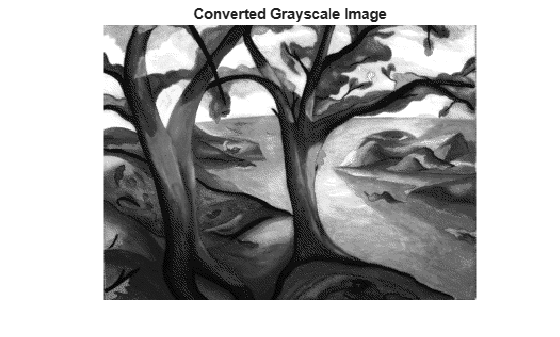# ind2gray

Convert indexed image to grayscale image

## Syntax

``I = ind2gray(X,cmap)``

## Description

example

````I = ind2gray(X,cmap)` converts the indexed image `X` with colormap `cmap` to a grayscale image, `I`. The `ind2gray` function removes the hue and saturation information from the input image while retaining the luminance.```

## Examples

collapse all

Load an indexed image into the workspace.

`[X, map] = imread('trees.tif');`

Convert the image to grayscale using `ind2gray`.

`I = ind2gray(X,map);`

Display the indexed image and the converted grayscale image.

```imshow(X,map) title('Indexed Image')``````figure imshow(I) title('Converted Grayscale Image')```## Input Arguments

collapse all

Indexed image, specified as a numeric array of any size and dimensionality.

Data Types: `single` | `double` | `uint8` | `uint16`

Colormap associated with indexed image `X`, specified as a c-by-3 numeric matrix with values in the range [0, 1]. Each row is a three-element RGB triplet that specifies the red, green, and blue components of a single color of the colormap.

Data Types: `double`

## Output Arguments

collapse all

Grayscale image, specified as a numeric array. `I` has the same size, dimensionality and class as `X`.

## Algorithms

`ind2gray` converts the colormap to NTSC coordinates using `rgb2ntsc`, and sets the hue and saturation components (I and Q) to zero, creating a gray colormap. `ind2gray` then replaces the indices in the image `X` with the corresponding grayscale intensity values in the gray colormap.

## Version History

Introduced before R2006a